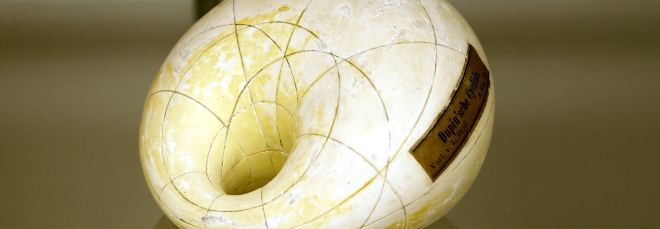## “Counting meromorphic differentials with zero residues on the Riemann sphere and the KP hierarchy”

Mercoledì 1 Giugno 2022, ore 14:30 - Aula 2BC30 - Alexandr Buryak (Higher School of Economics, Moscow)

Abstract

Let us fix n integers (multiplicities) and consider the set of configurations of n points on the sphere such that there exists a meromorphic differential with zero residues and with the divisor given by the linear combination of the n points with the prescribed multiplicities. Let us factorize this set by the automorphism group of the sphere. The resulting set is finite if and only if the number of poles is equal to n-2. The cardinalities of these sets can be considered as natural analogs of Hurwitz numbers. In our joint work with Paolo Rossi and Dimitri Zvonkine we proved that these numbers are exactly the coefficients in the equations of the dispersionless KP hierarchy.